# ICSE Class 10 Chemistry Question Paper Solution 2015

Just solving the Chemistry question paper will not give any fruitful result to students. To get the maximum benefit of solving the previous years Chemistry papers, students must check their answer sheet by themselves and should find out their mistakes. Doing, so will help them in identifying the loopholes in their exam preparation and will also improve their performance for the exam. So, go through the ICSE Class 10 Chemistry Question Paper 2015 Solution and leave no stone unturned in the board exam preparation.

The ICSE Class 10 Chemistry 2015 exam was conducted for 2 hours of time duration. Here we have provided the complete ICSE Class 10 Chemistry Question Paper Solution 2015 for free downloading to students.

#### Students can have a look at the ICSE Class 10 Chemistry Question Paper Solution 2015 below: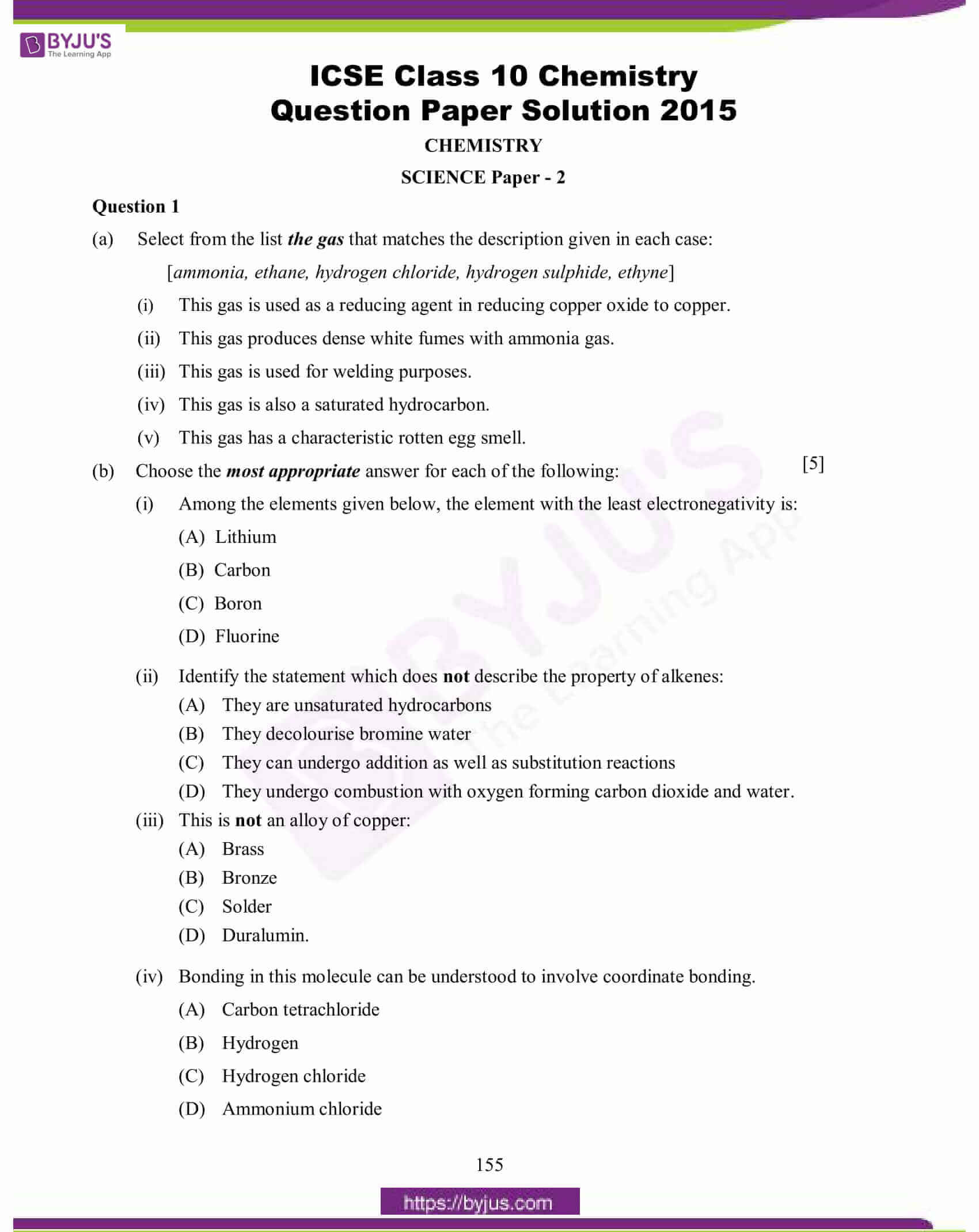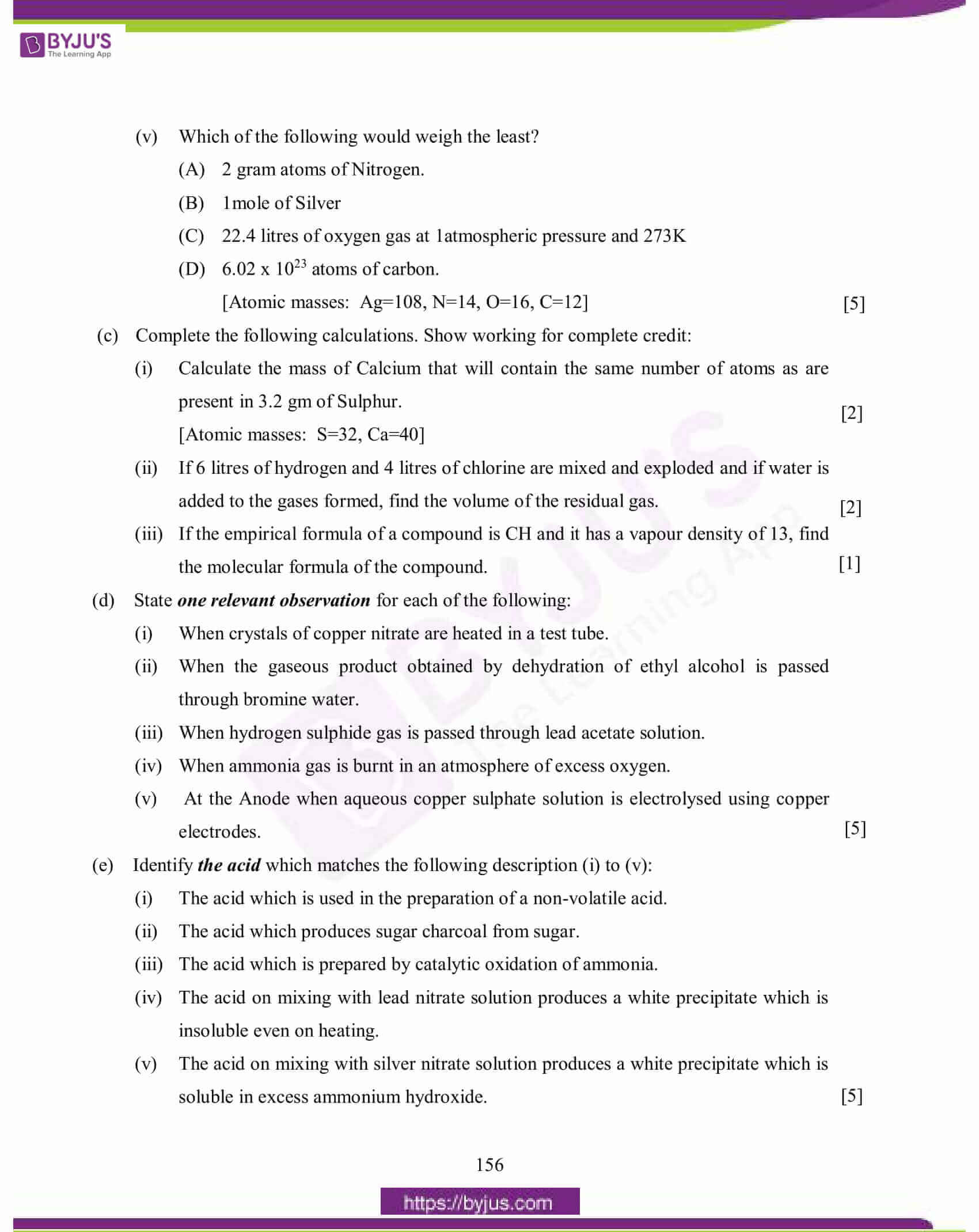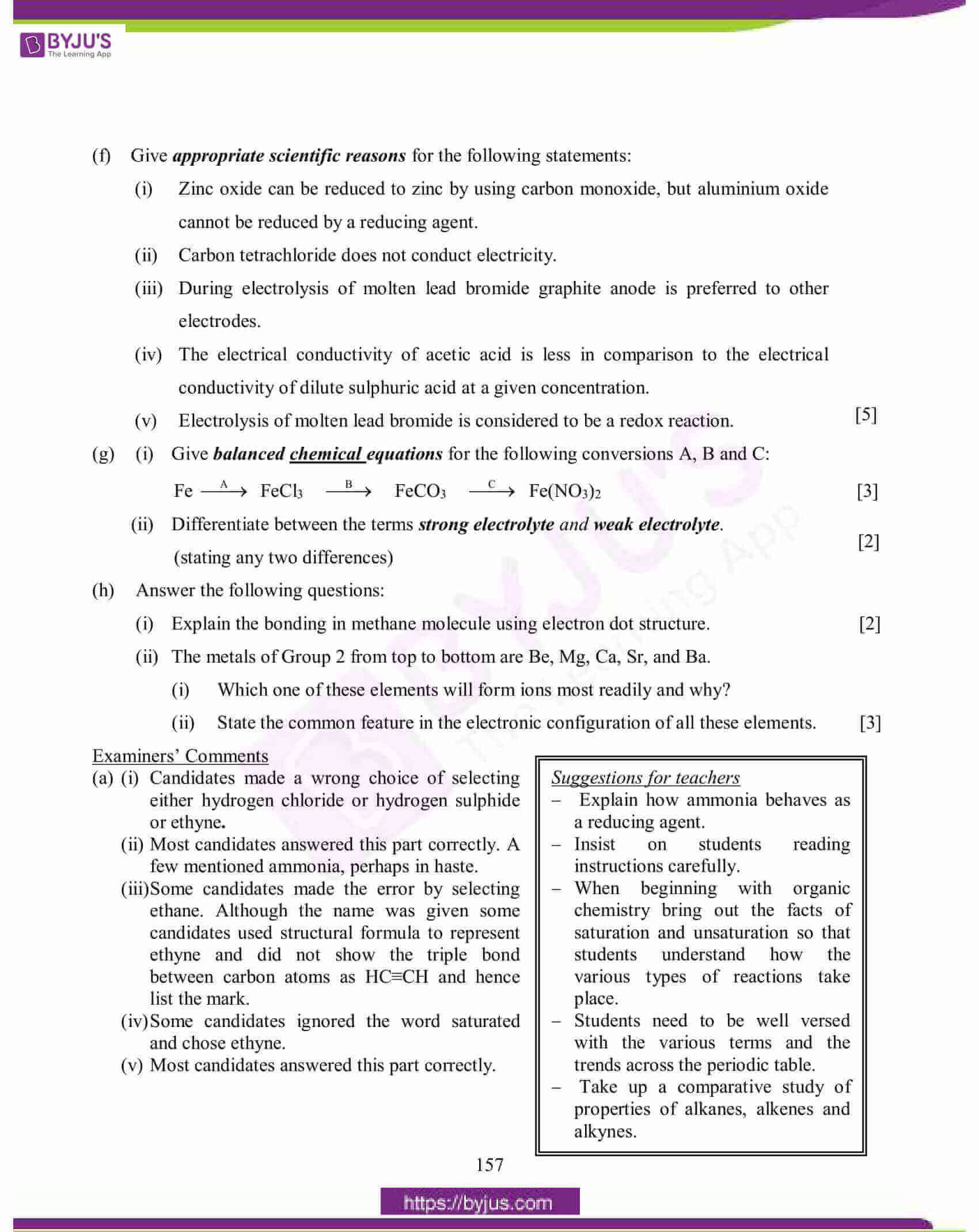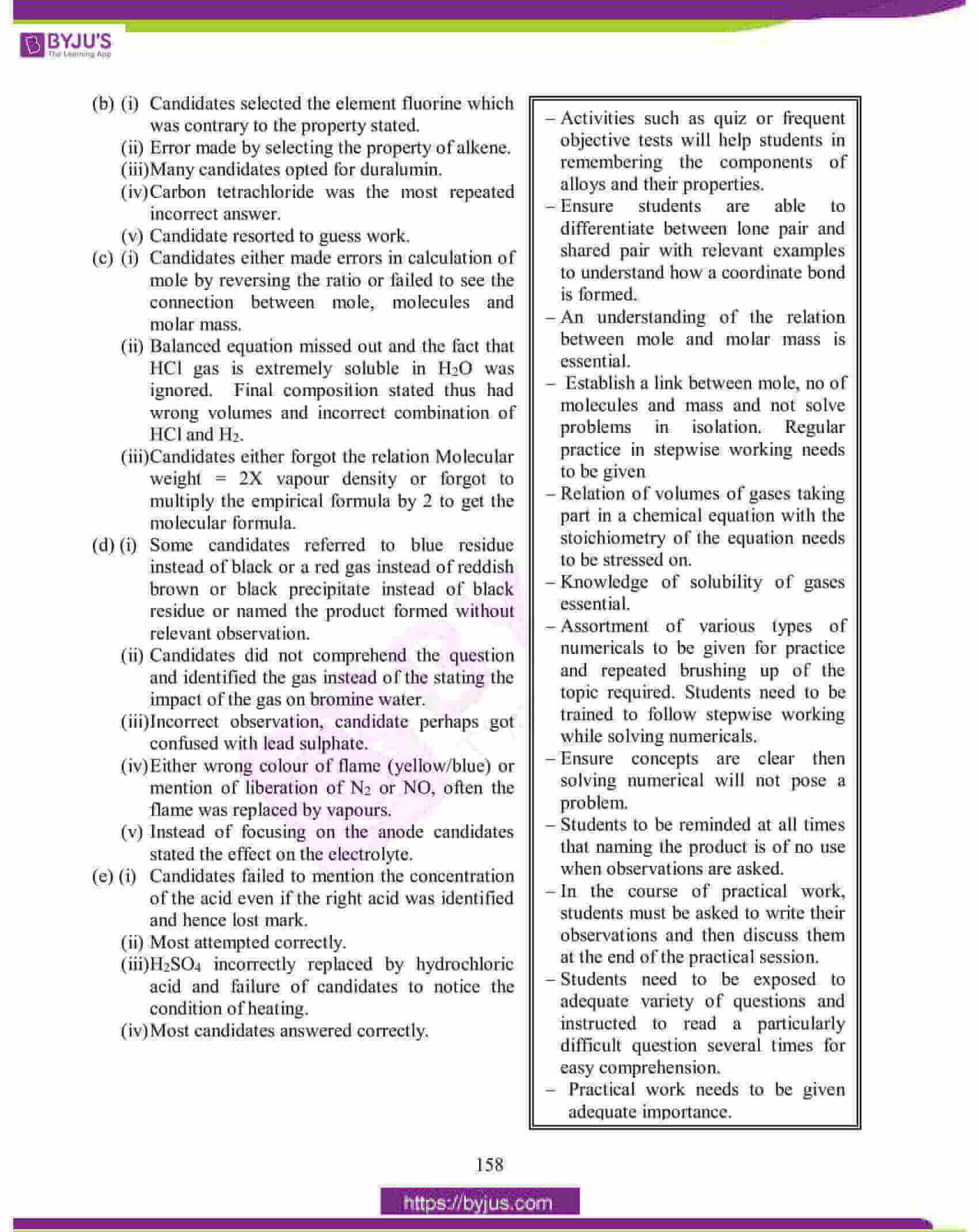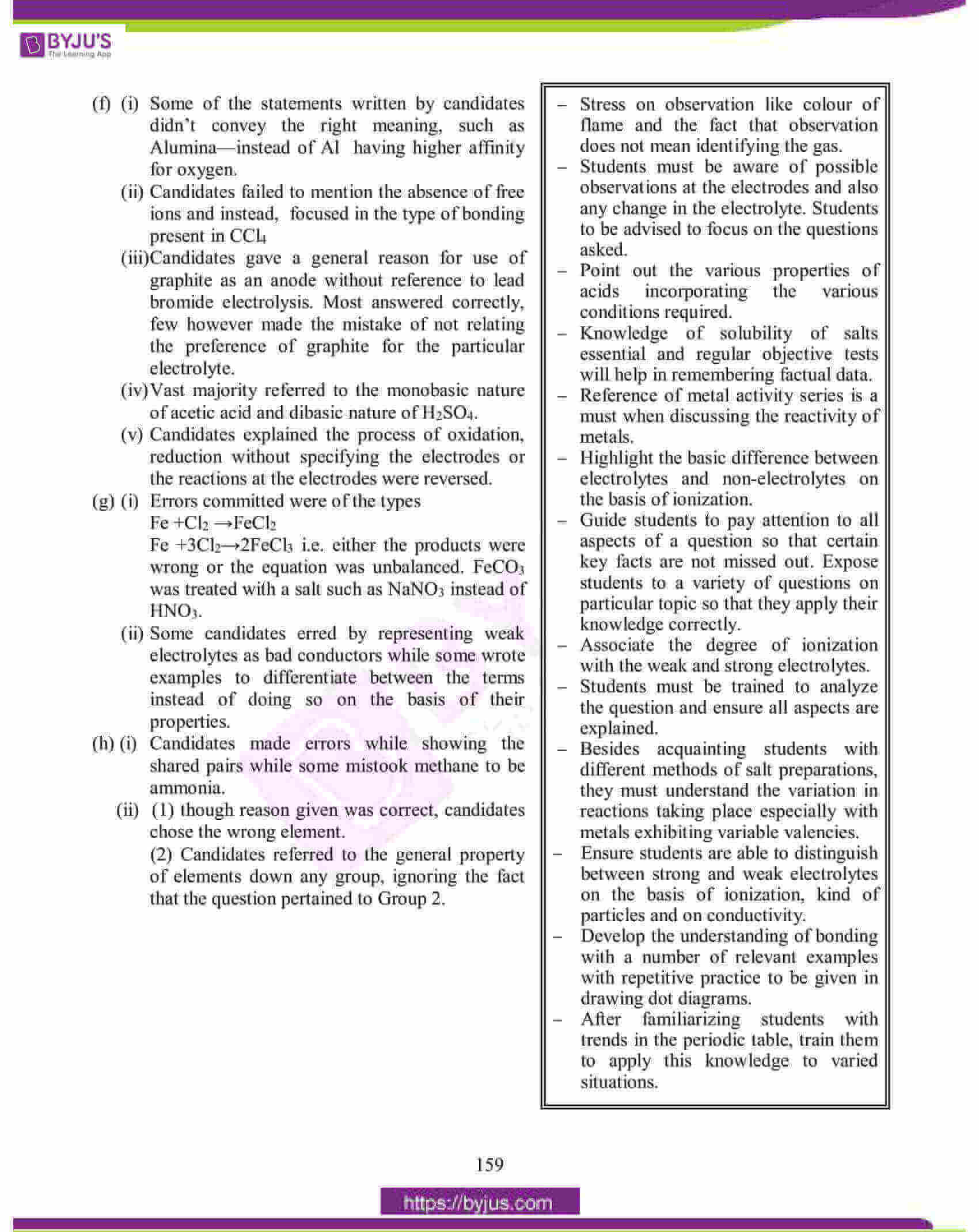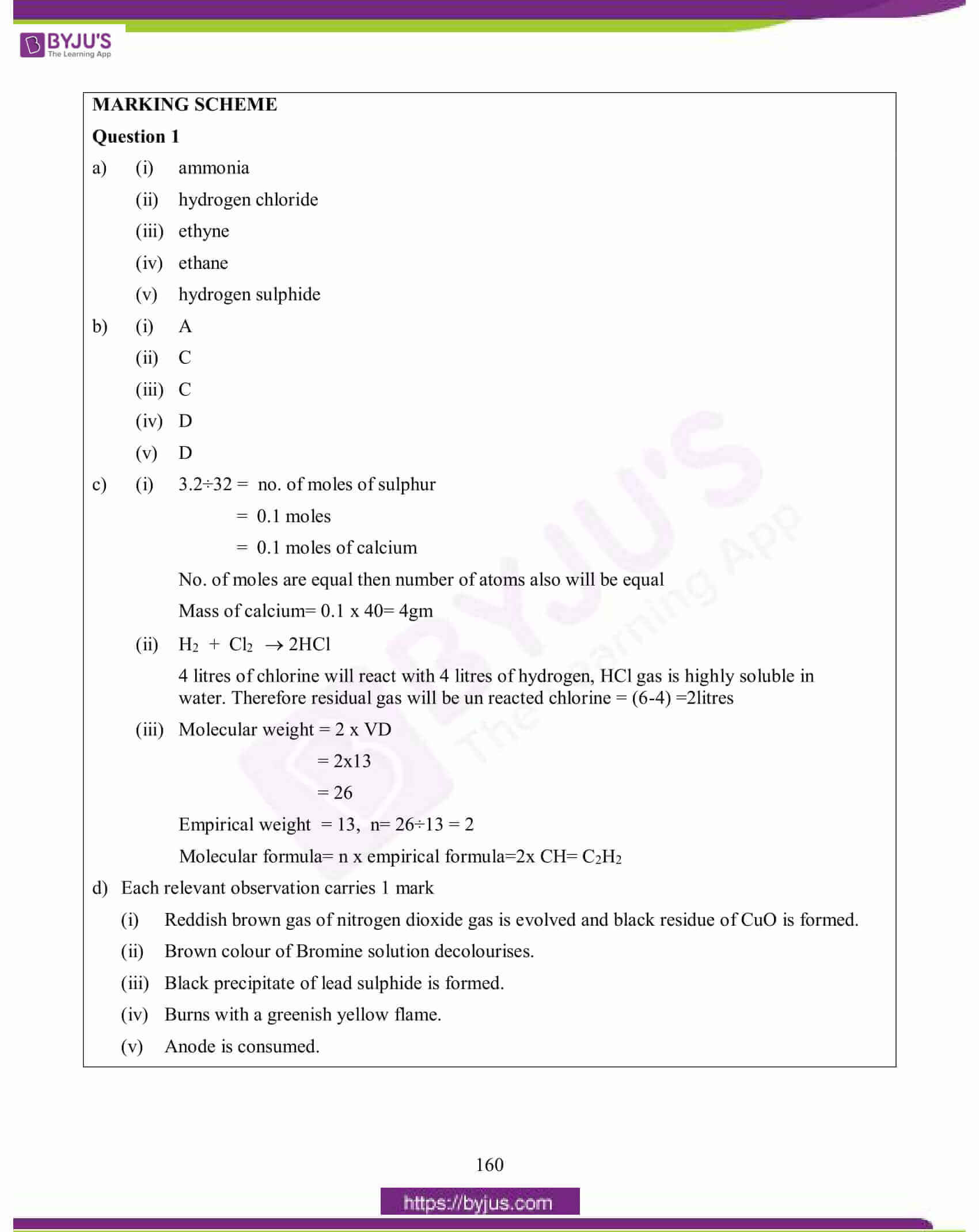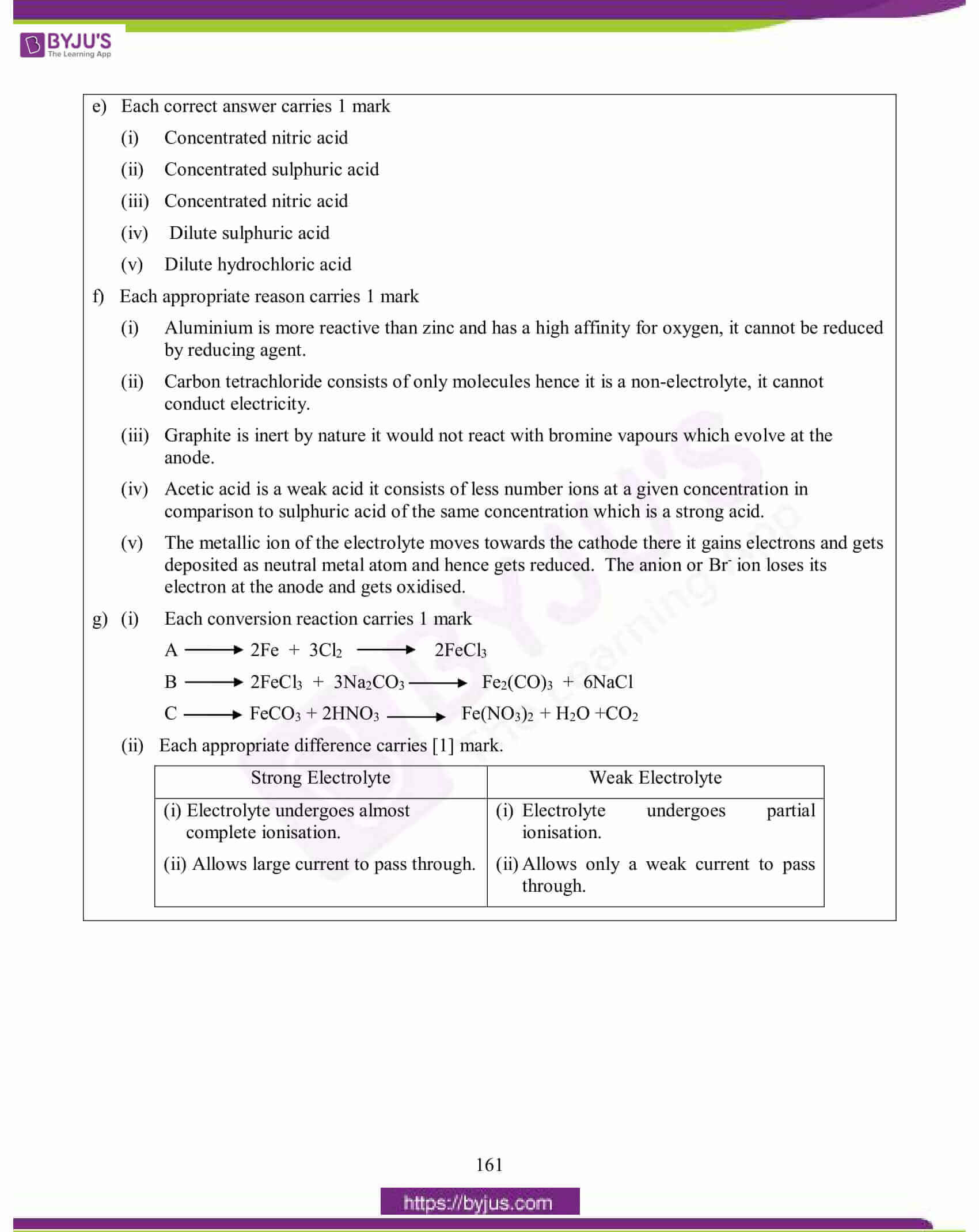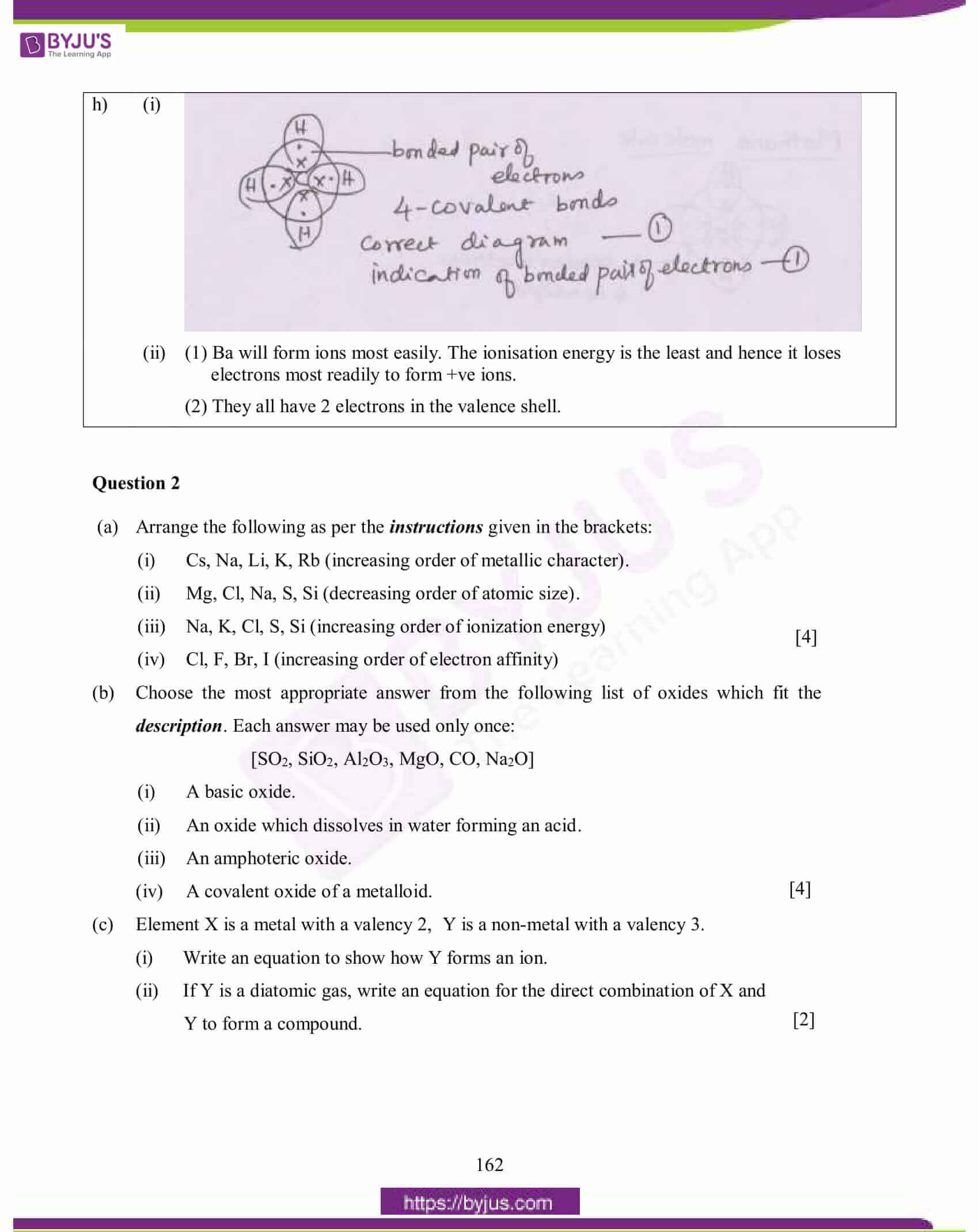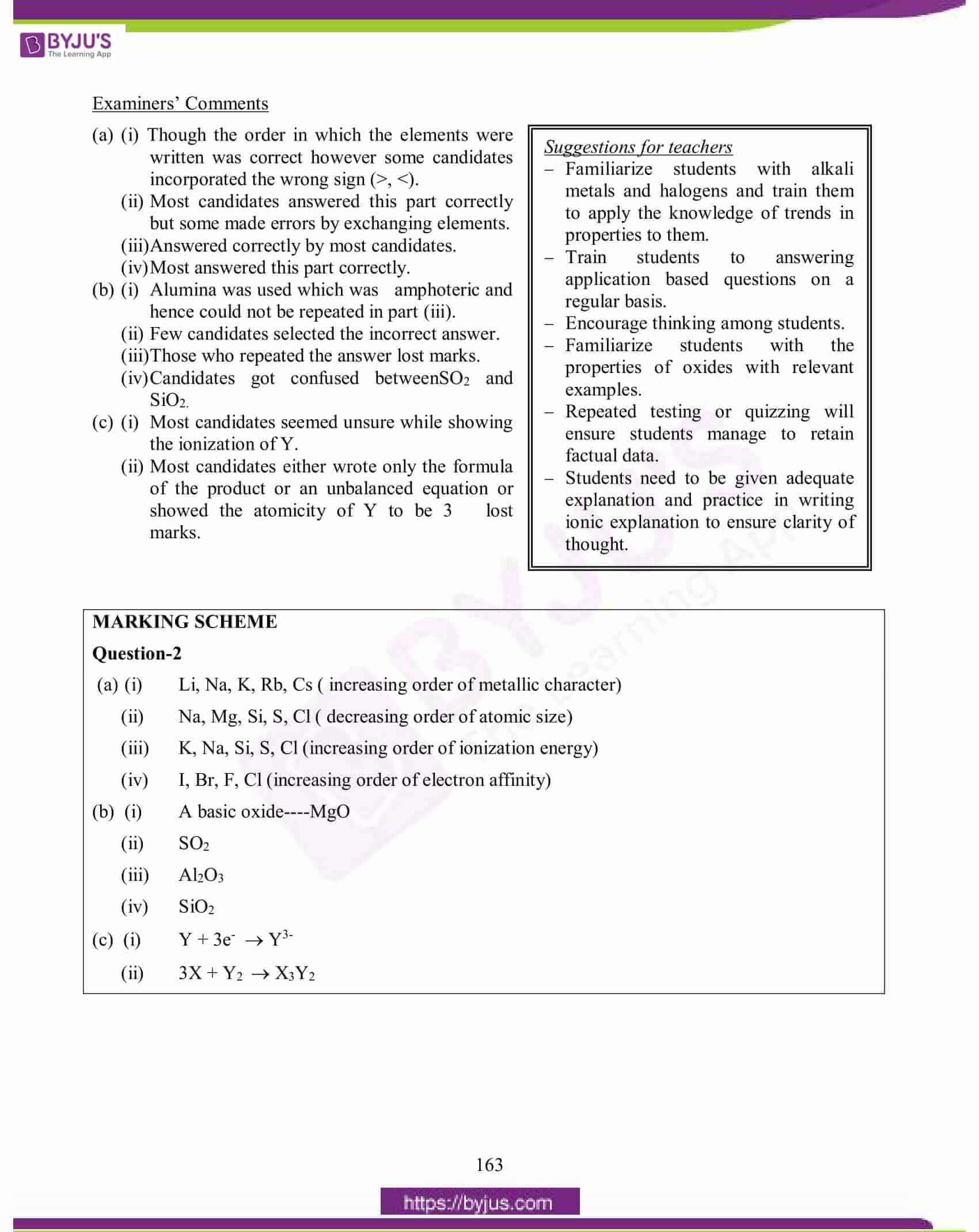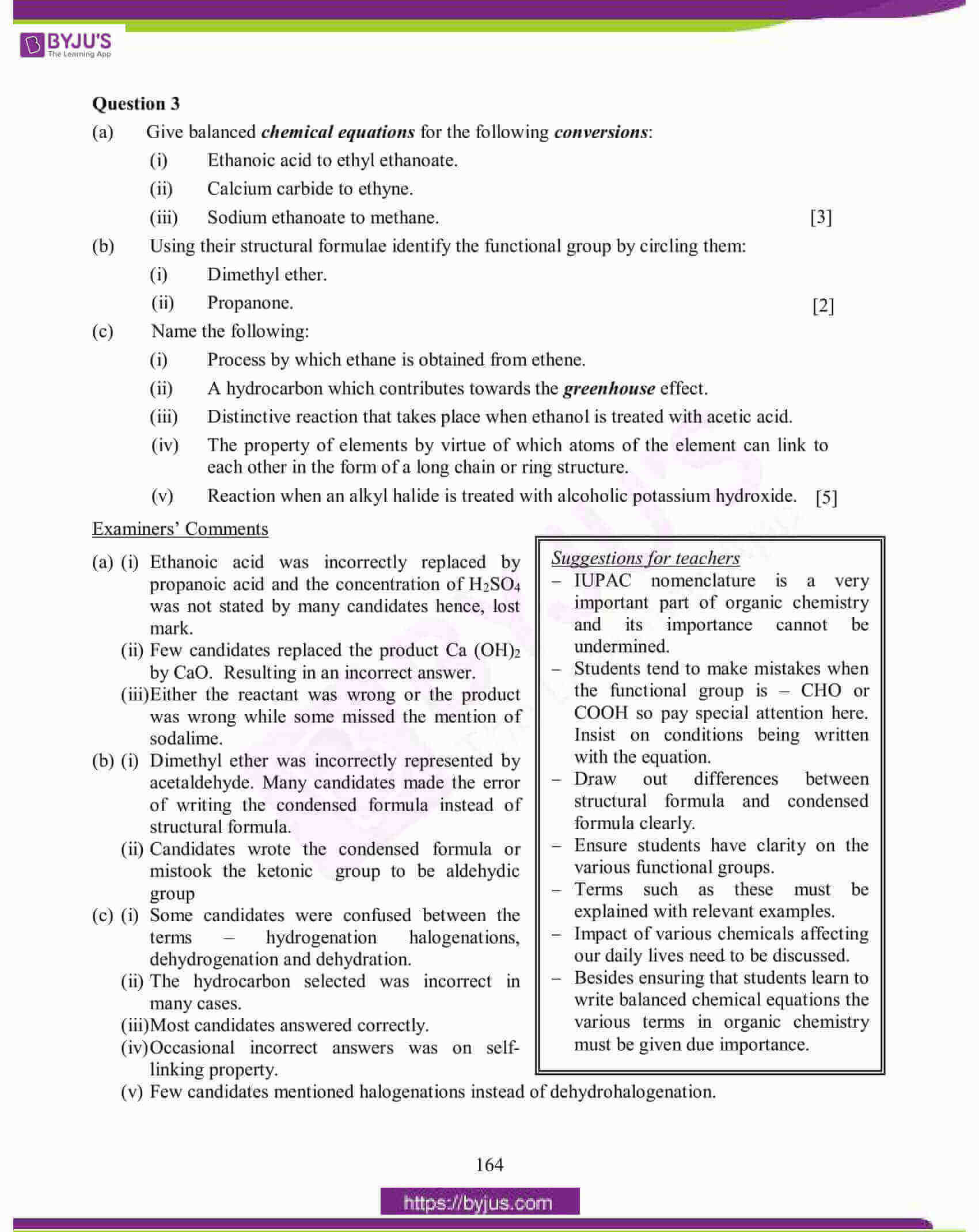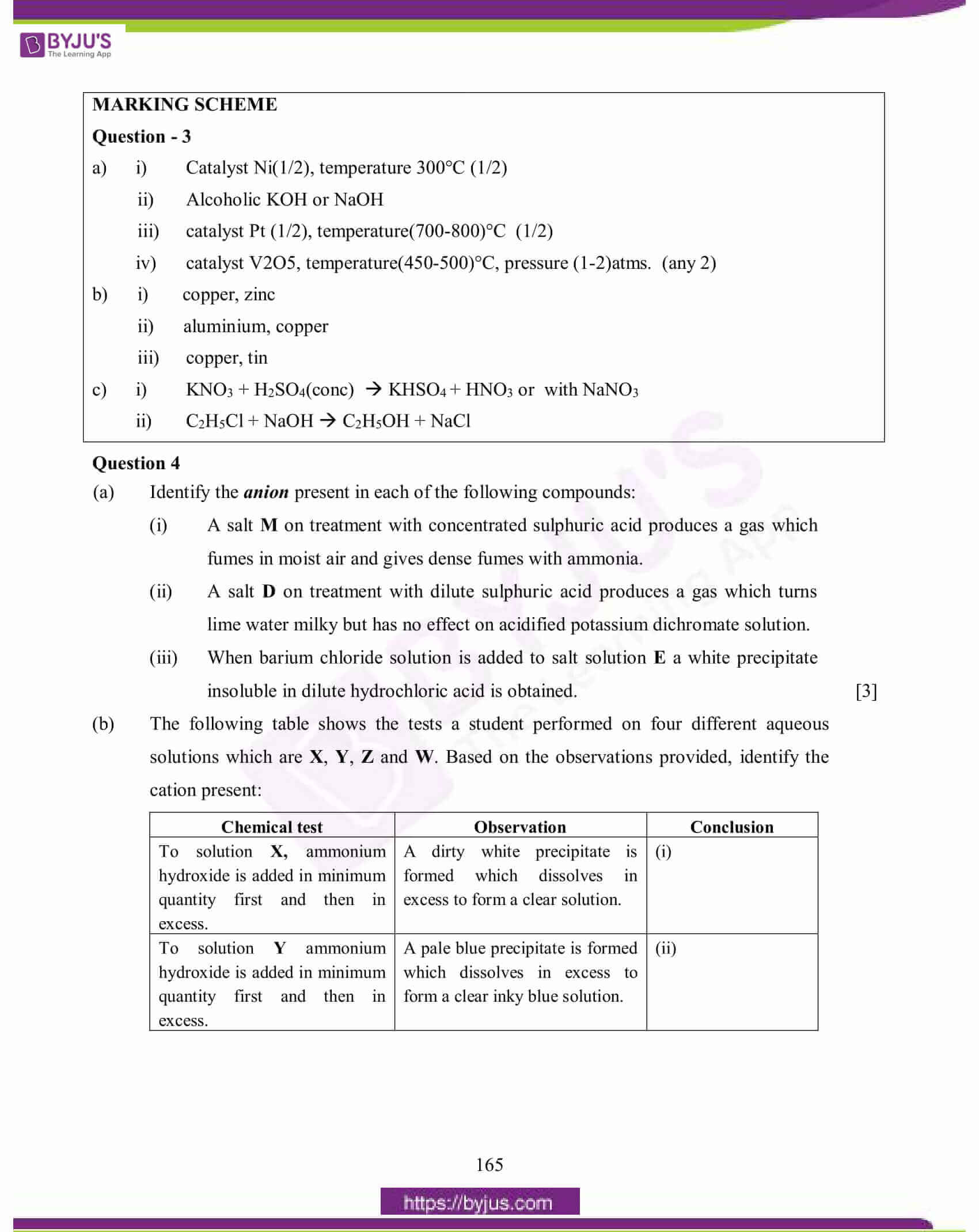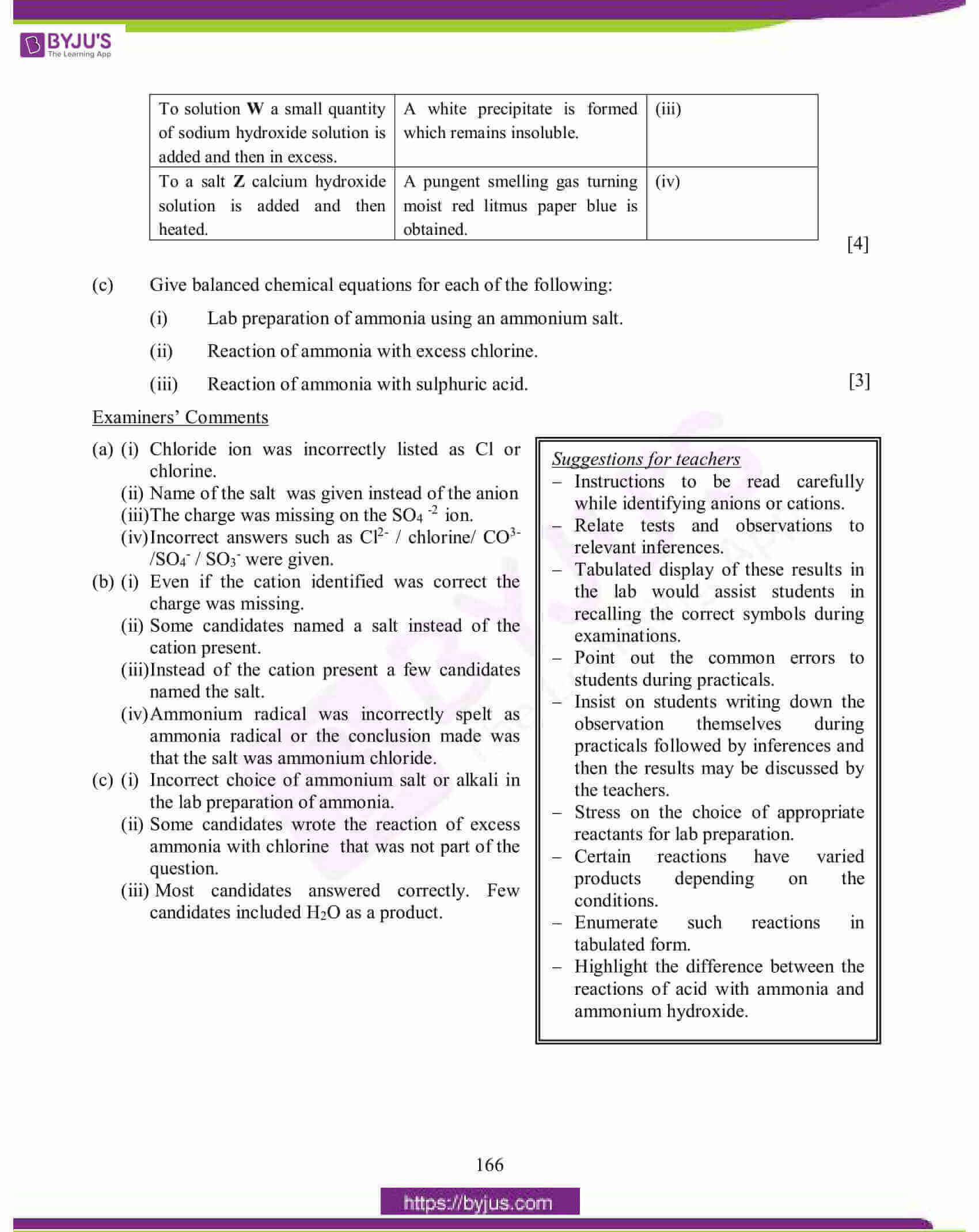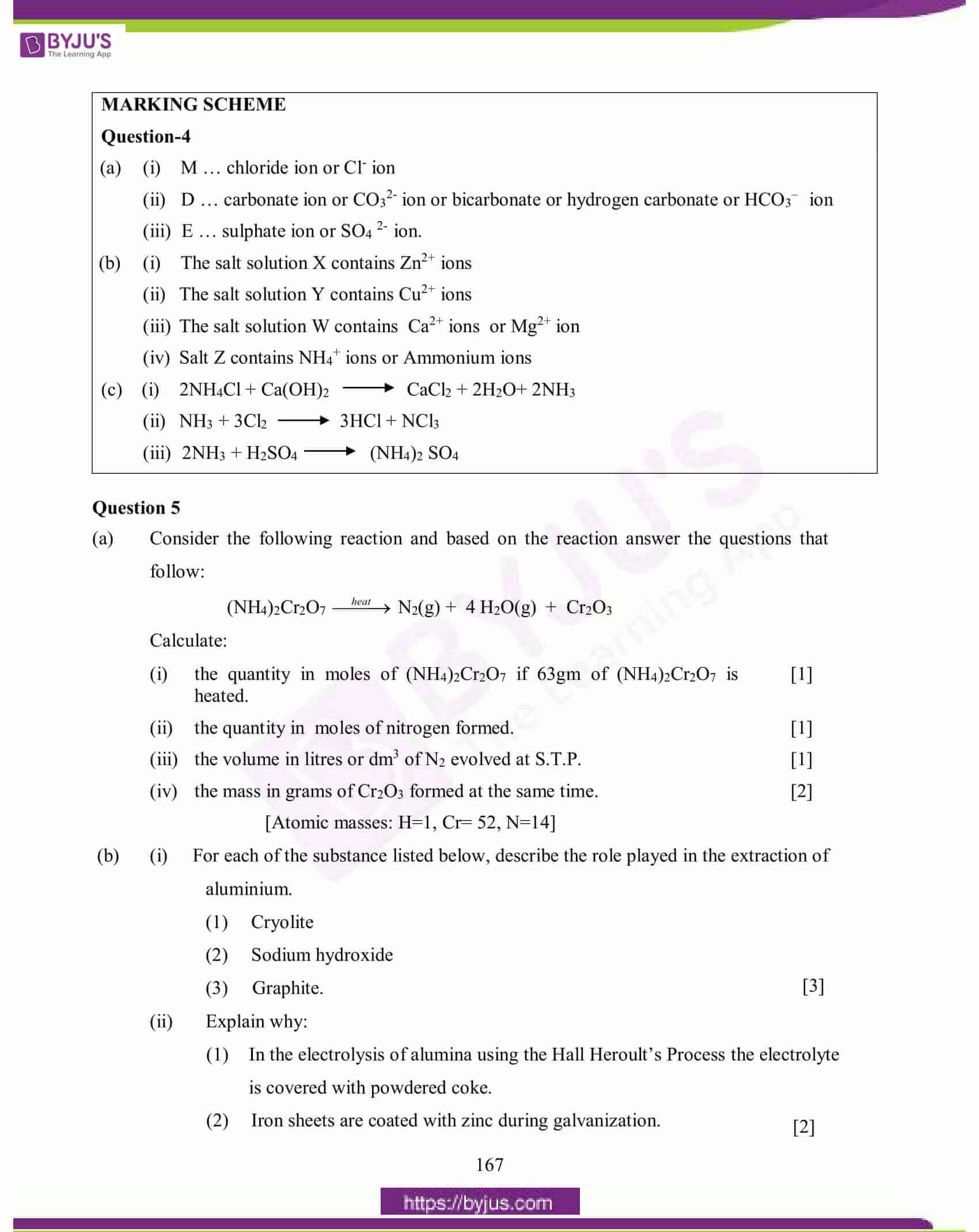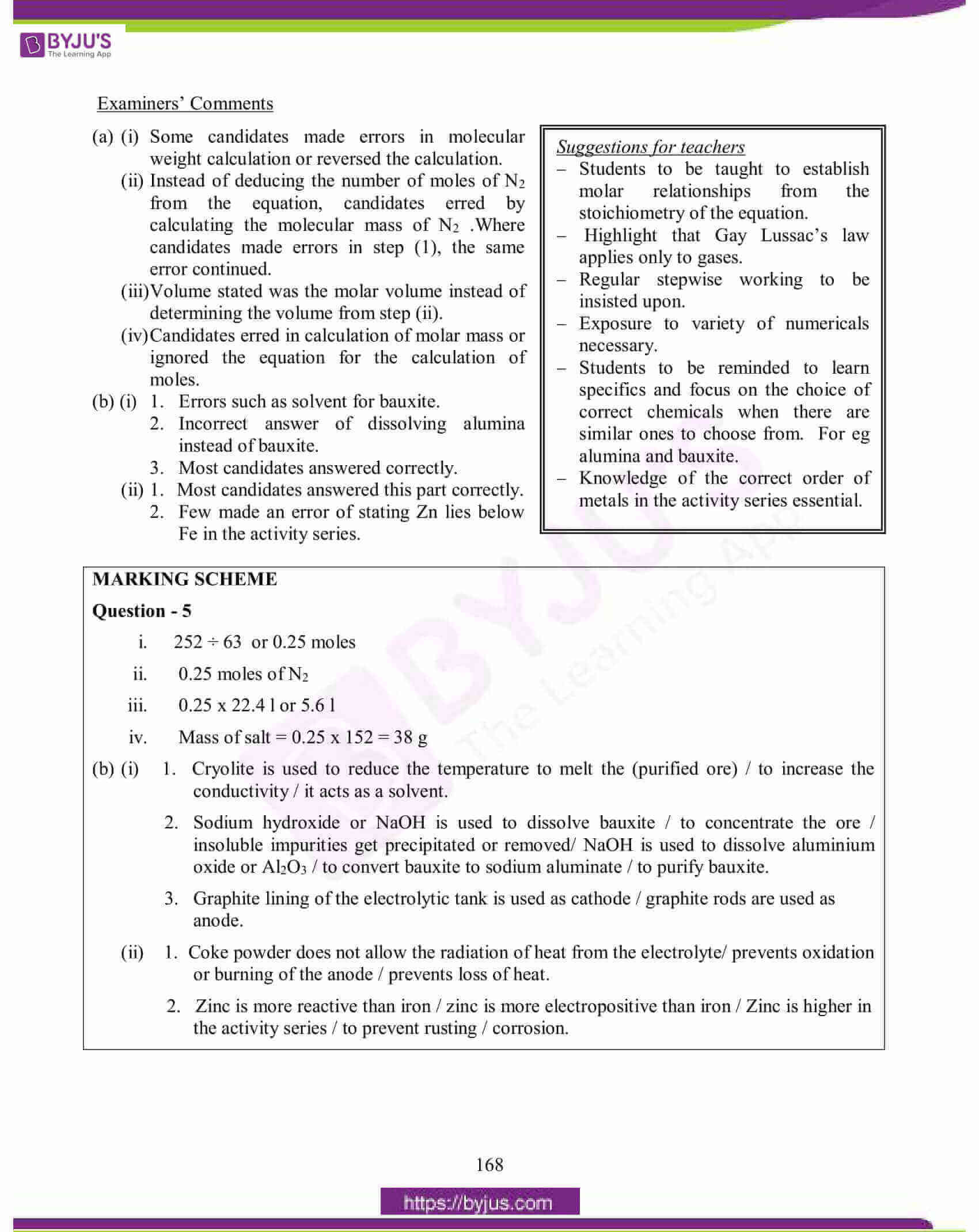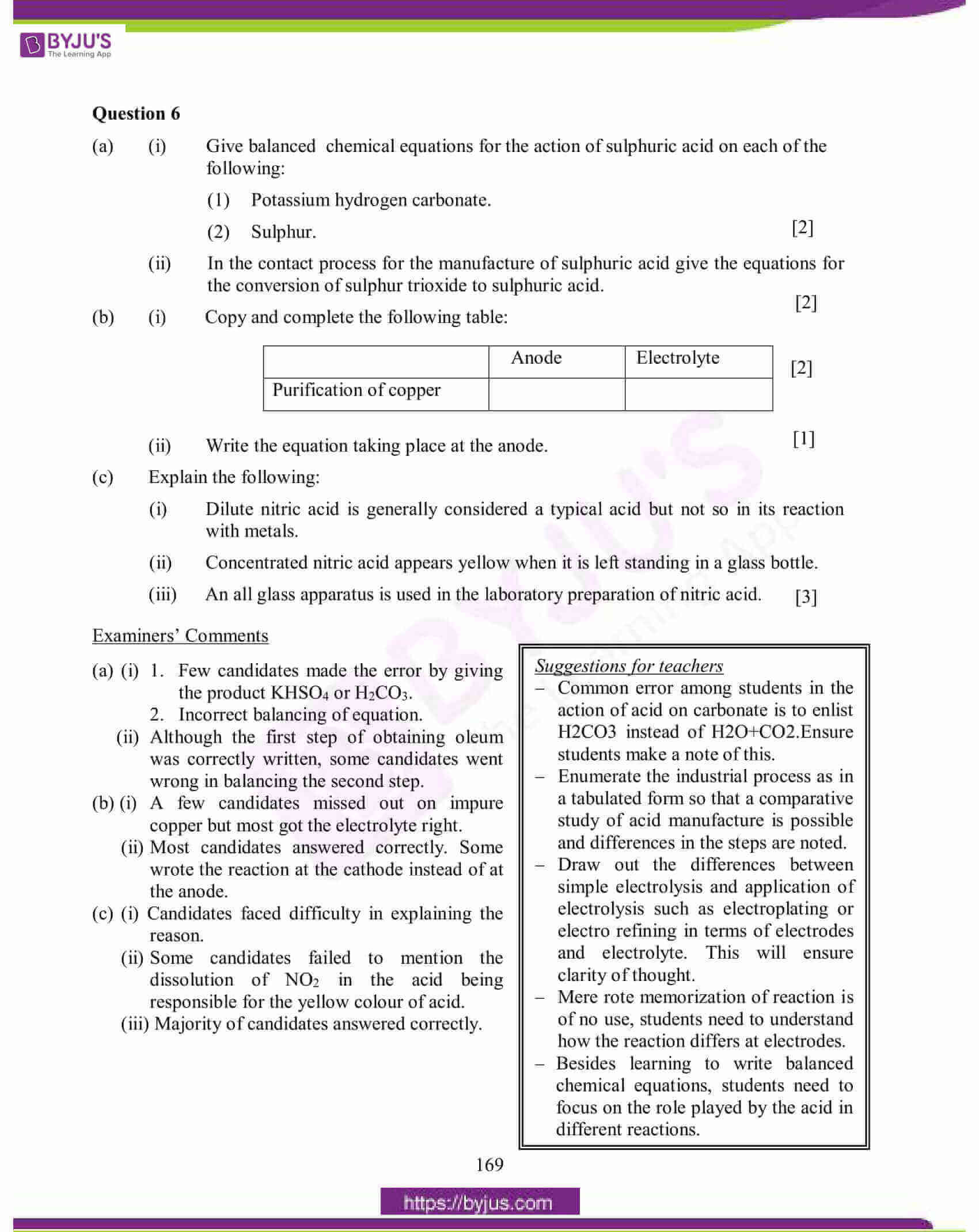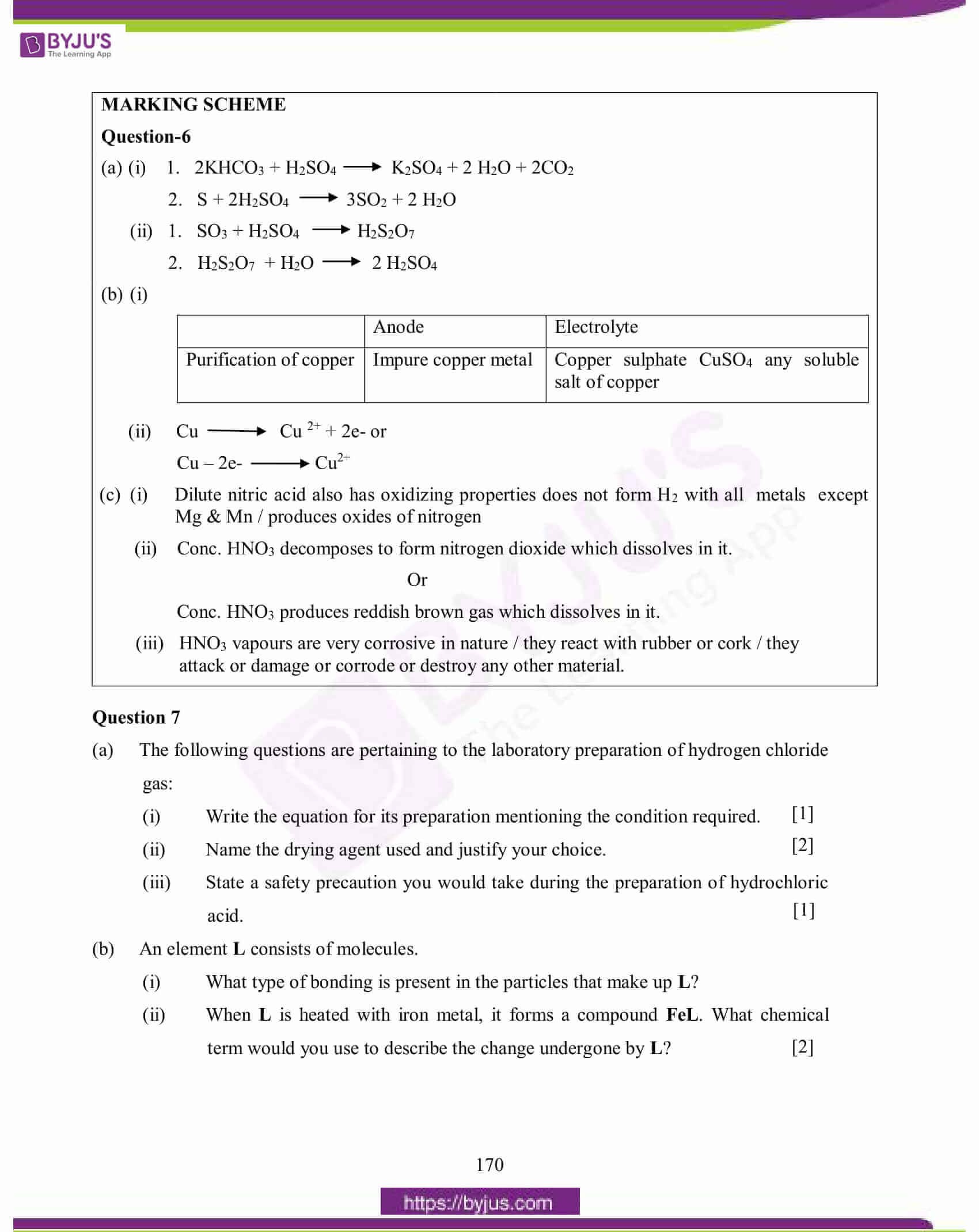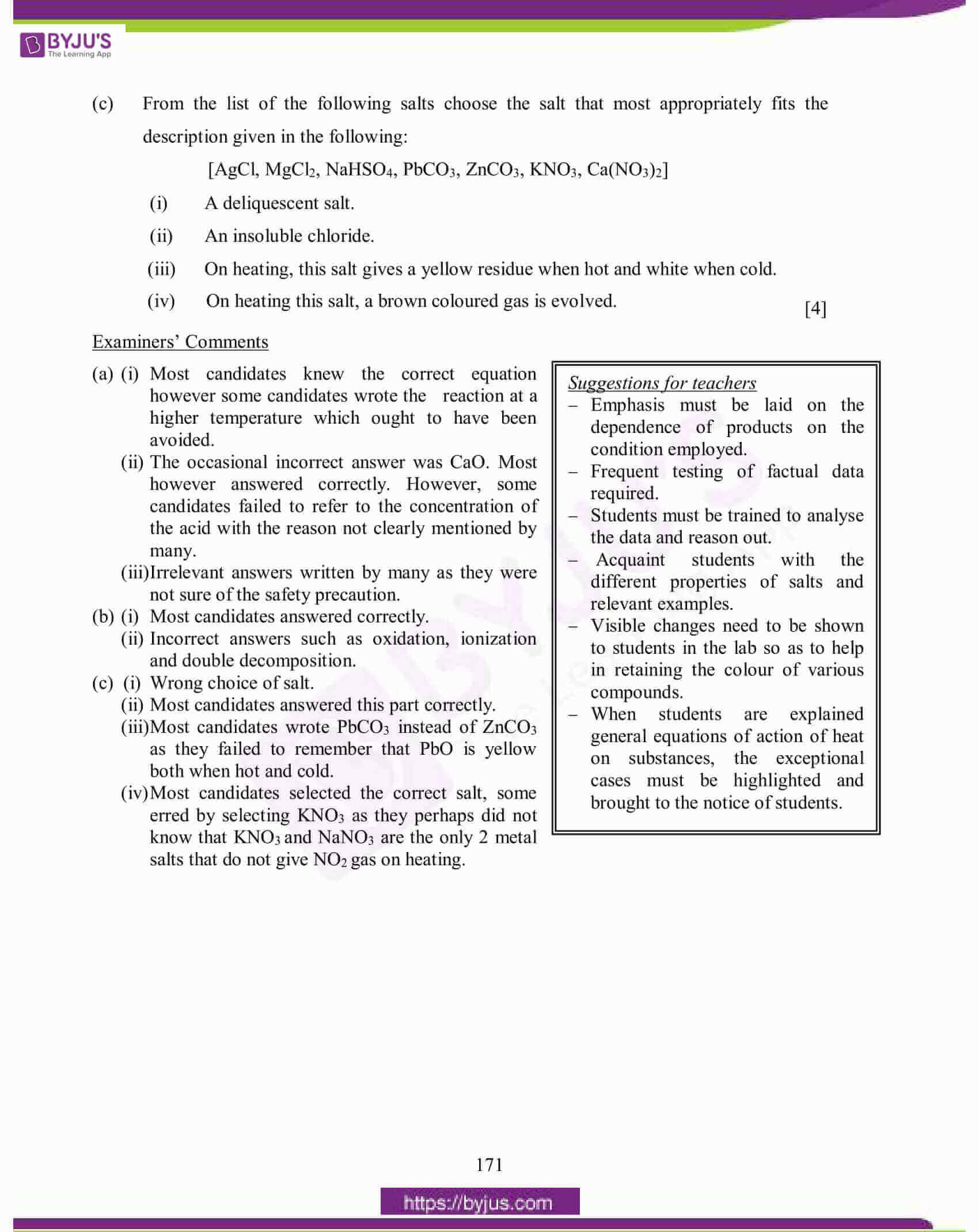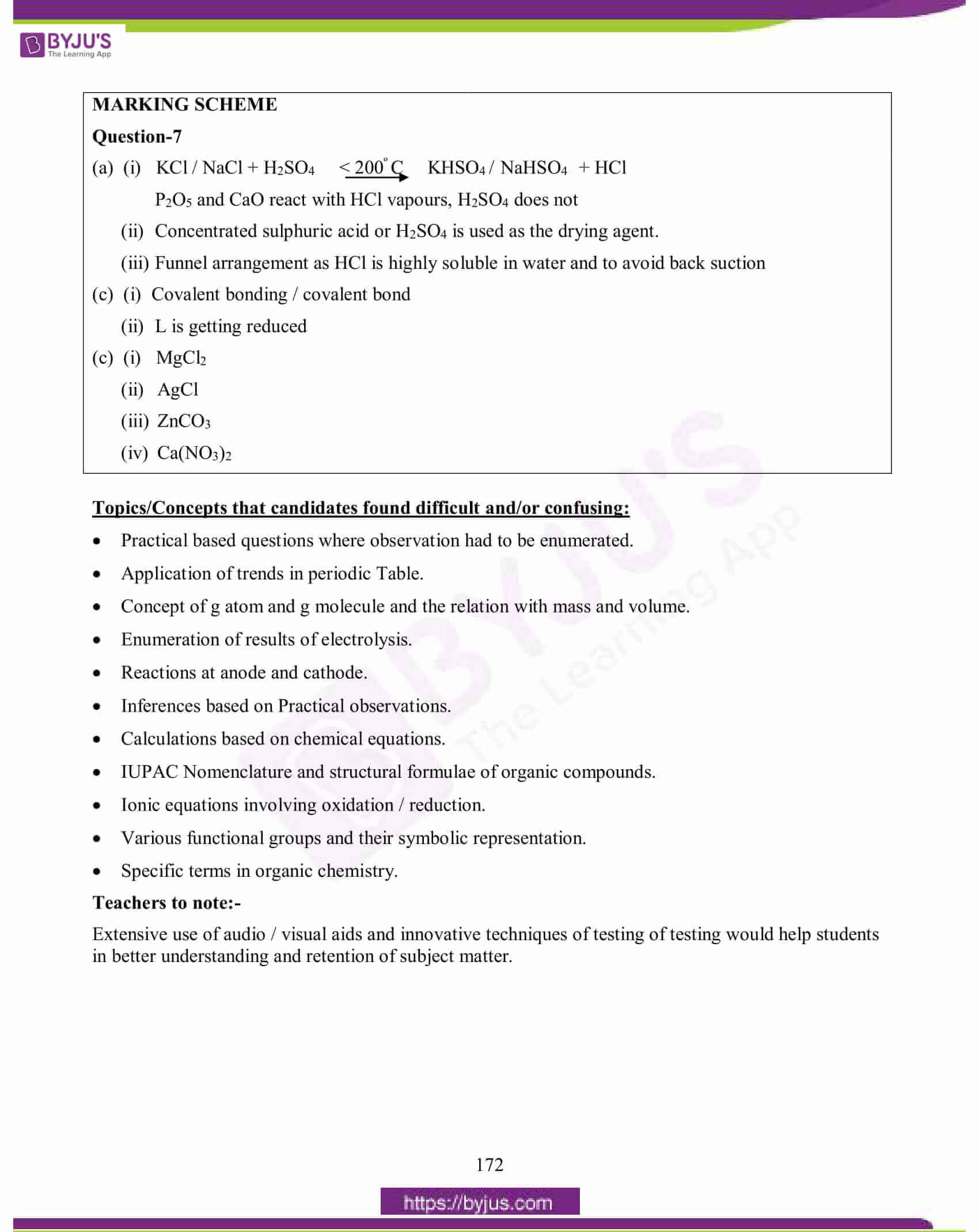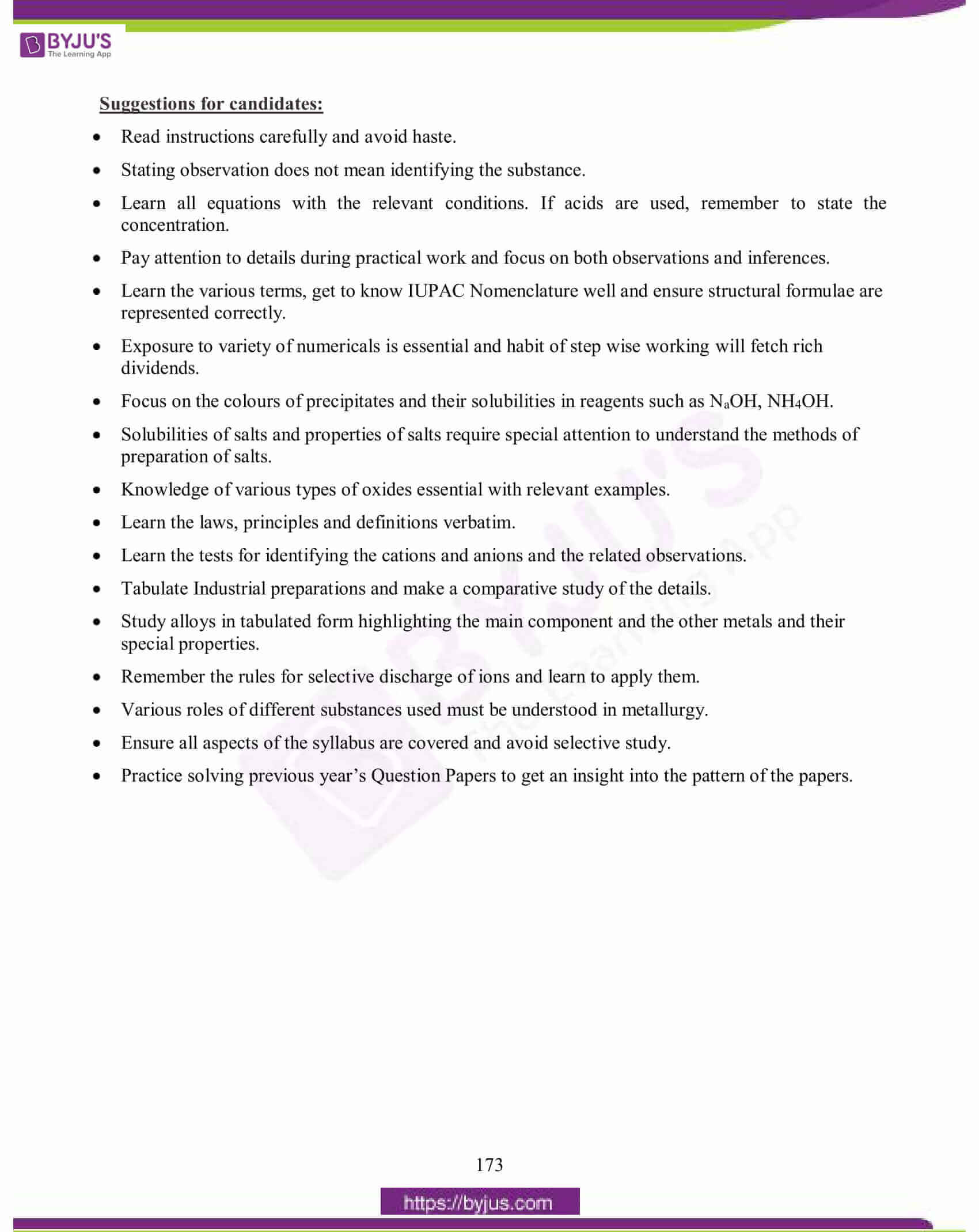### Topics Found Confusing and Difficult in Chemistry 2015 Question Paper

Students can have an overview of the difficult and confusing topics of 2015 Chemistry Science Paper 2.

• Practical based questions where observation had to be enumerated.
• Application of trends in periodic Table.
• Concept of g atom and g molecule and the relation with mass and volume.
• Enumeration of results of electrolysis.
• Reactions at anode and cathode.
• Inferences based on Practical observations.
• Calculations based on chemical equations.
• IUPAC Nomenclature and structural formulae of organic compounds.
• Ionic equations involving oxidation / reduction.
• Various functional groups and their symbolic representation.
• Specific terms in organic chemistry.

## ICSE Class 10 Chemistry (Science Paper 2) Question Paper 2015 With Solution

### Question 1:

(a) Select from the list the gas that matches the description given in each case:

[ammonia, ethane, hydrogen chloride, hydrogen sulphide, ethyne]

(i) This gas is used as a reducing agent in reducing copper oxide to copper.

(ii) This gas produces dense white fumes with ammonia gas.

(iii) This gas is used for welding purposes.

(iv) This gas is also a saturated hydrocarbon.

(v) This gas has a characteristic rotten egg smell.

(b) Choose the most appropriate answer for each of the following:

(i) Among the elements given below, the element with the least electronegativity is:

(A) Lithium

(B) Carbon

(C) Boron

(D) Fluorine

(ii) Identify the statement which does not describe the property of alkenes:

(A) They are unsaturated hydrocarbons

(B) They decolourise bromine water

(C) They can undergo addition as well as substitution reactions

(D) They undergo combustion with oxygen forming carbon dioxide and water.

(iii) This is not an alloy of copper:

(A) Brass

(B) Bronze

(C) Solder

(D) Duralumin.

(iv) Bonding in this molecule can be understood to involve coordinate bonding.

(A) Carbon tetrachloride

(B) Hydrogen

(C) Hydrogen chloride

(D) Ammonium chloride

(v) Which of the following would weigh the least?

(A) 2 gram atoms of Nitrogen.

(B) 1mole of Silver

(C) 22.4 litres of oxygen gas at 1atmospheric pressure and 273K

(D) 6.02 x 1023 atoms of carbon.

[Atomic masses: Ag=108, N=14, O=16, C=12]

(c) Complete the following calculations. Show working for complete credit:

(i) Calculate the mass of Calcium that will contain the same number of atoms as are present in 3.2 gm of Sulphur. [Atomic masses: S=32, Ca=40]

(ii) If 6 litres of hydrogen and 4 litres of chlorine are mixed and exploded and if water is added to the gases formed, find the volume of the residual gas.

(iii) If the empirical formula of a compound is CH and it has a vapour density of 13, find the molecular formula of the compound.

(d) State one relevant observation for each of the following:

(i) When crystals of copper nitrate are heated in a test tube.

(ii) When the gaseous product obtained by dehydration of ethyl alcohol is passed through bromine water.

(iii) When hydrogen sulphide gas is passed through lead acetate solution.

(iv) When ammonia gas is burnt in an atmosphere of excess oxygen.

(v) At the Anode when aqueous copper sulphate solution is electrolysed using copper electrodes.

(e) Identify the acid which matches the following description (i) to (v):

(i) The acid which is used in the preparation of a non-volatile acid.

(ii) The acid which produces sugar charcoal from sugar.

(iii) The acid which is prepared by catalytic oxidation of ammonia.

(iv) The acid on mixing with lead nitrate solution produces a white precipitate which is insoluble even on heating.

(v) The acid on mixing with silver nitrate solution produces a white precipitate which is soluble in excess ammonium hydroxide.

(f) Give appropriate scientific reasons for the following statements:

(i) Zinc oxide can be reduced to zinc by using carbon monoxide, but aluminium oxide cannot be reduced by a reducing agent.

(ii) Carbon tetrachloride does not conduct electricity.

(iii) During electrolysis of molten lead bromide graphite anode is preferred to other electrodes.

(iv) The electrical conductivity of acetic acid is less in comparison to the electrical conductivity of dilute sulphuric acid at a given concentration.

(v) Electrolysis of molten lead bromide is considered to be a redox reaction.

(g) (i) Give balanced chemical equations for the following conversions A, B and C: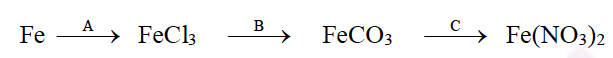(ii) Differentiate between the terms strong electrolyte and weak electrolyte. (stating any two differences)

(i) Explain the bonding in methane molecules using electron dot structure.

(ii) The metals of Group 2 from top to bottom are Be, Mg, Ca, Sr, and Ba.

(i) Which one of these elements will form ions most readily and why?

(ii) State the common feature in the electronic configuration of all these element

a) (i) ammonia

(ii) hydrogen chloride

(iii) ethyne

(iv) ethane

(v) hydrogen sulphide

b) (i) A

(ii) C

(iii) C

(iv) D

(v) D

c) (i) 3.2÷32 = no. of moles of sulphur

= 0.1 moles

= 0.1 moles of calcium

No. of moles are equal then number of atoms also will be equal

Mass of calcium= 0.1 x 40= 4gm

(ii) H2 + Cl2 → 2HCl

4 litres of chlorine will react with 4 litres of hydrogen, HCl gas is highly soluble in water. Therefore residual gas will be un reacted chlorine = (6 – 4) = 2 litres

(iii) Molecular weight = 2 x VD

= 2 x 13

= 26

Empirical weight = 13, n = 26 ÷ 13 = 2

Molecular formula= n x empirical formula=2 x CH= C2H2

d) Each relevant observation carries 1 mark

(i) Reddish brown gas of nitrogen dioxide gas is evolved and black residue of CuO is formed.

(ii) Brown colour of Bromine solution decolourises.

(iii) Black precipitate of lead sulphide is formed.

(iv) Burns with a greenish yellow flame.

(v) Anode is consumed.

e) Each correct answer carries 1 mark

(i) Concentrated nitric acid

(ii) Concentrated sulphuric acid

(iii) Concentrated nitric acid

(iv) Dilute sulphuric acid

(v) Dilute hydrochloric acid

f) Each appropriate reason carries 1 mark

(i) Aluminium is more reactive than zinc and has a high affinity for oxygen, it cannot be reduced by reducing agent.

(ii) Carbon tetrachloride consists of only molecules hence it is a non-electrolyte, it cannot conduct electricity.

(iii) Graphite is inert by nature it would not react with bromine vapours which evolve at the anode.

(iv) Acetic acid is a weak acid it consists of less number ions at a given concentration in comparison to sulphuric acid of the same concentration which is a strong acid.

(v) The metallic ion of the electrolyte moves towards the cathode there it gains electrons and gets deposited as neutral metal atom and hence gets reduced. The anion or Brion loses its electron at the anode and gets oxidised.

g) (i) Each conversion reaction carries 1 mark

A → 2Fe + 3Cl2 → 2FeCl3

B → 2FeCl3 + 3Na2CO3 → Fe2(CO)3 + 6NaCl

C → FeCO3 + 2HNO3 → Fe(NO3)2 + H2O +CO2

(ii) Each appropriate difference carries  mark.

 Strong Electrolyte Weak Electrolyte (i) Electrolyte undergoes almost complete ionisation. (i) Electrolyte undergoes partial ionisation. (ii) Allows large current to pass through. (ii) Allows only a weak current to pass through.

h) (i)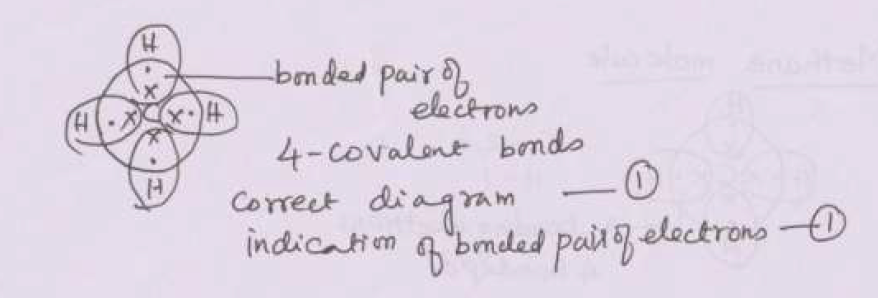(ii)

1. Ba will form ions most easily. The ionisation energy is the least and hence it loses electrons most readily to form +ve ions.
2. They all have 2 electrons in the valence shell

### Question 2:

(a) Arrange the following as per the instructions given in the brackets:

(i) Cs, Na, Li, K, Rb (increasing order of metallic character).

(ii) Mg, Cl, Na, S, Si (decreasing order of atomic size).

(iii) Na, K, Cl, S, Si (increasing order of ionization energy)

(iv) Cl, F, Br, I (increasing order of electron affinity)

(b) Choose the most appropriate answer from the following list of oxides which fit the description. Each answer may be used only once:

[SO2, SiO2, Al2O3, MgO, CO, Na2O]

(i) A basic oxide.

(ii) An oxide which dissolves in water forming an acid.

(iii) An amphoteric oxide.

(iv) A covalent oxide of a metalloid.

(c) Element X is a metal with a valency 2, Y is a non-metal with a valency 3.

(i) Write an equation to show how Y forms an ion.

(ii) If Y is a diatomic gas, write an equation for the direct combination of X and Y to form a compound.

(a) (i) Li, Na, K, Rb, Cs ( increasing order of metallic character)

(ii) Na, Mg, Si, S, Cl ( decreasing order of atomic size)

(iii) K, Na, Si, S, Cl (increasing order of ionization energy)

(iv) I, Br, F, Cl (increasing order of electron affinity)

(b) (i) A basic oxide—-MgO

(ii) SO2

(iii) Al2O3

(iv) SiO2

(c) (i) Y + 3e → Y3-

(ii) 3X + Y2 → X3Y2

### Question 3:

(a) Give balanced chemical equations for the following conversions:

(i) Ethanoic acid to ethyl ethanoate.

(ii) Calcium carbide to ethyne.

(iii) Sodium ethanoate to methane.

(b) Using their structural formulae identify the functional group by circling them:

(i) Dimethyl ether.

(ii) Propanone.

(c) Name the following:

(i) Process by which ethane is obtained from ethene.

(ii) A hydrocarbon which contributes towards the greenhouse effect.

(iii) Distinctive reaction that takes place when ethanol is treated with acetic acid.

(iv) The property of elements by virtue of which atoms of the element can link to each other in the form of a long chain or ring structure.

(v) Reaction when an alkyl halide is treated with alcoholic potassium hydroxide.

a) i) Catalyst Ni(1/2), temperature 300°C (1/2)

ii) Alcoholic KOH or NaOH

iii) catalyst Pt (1/2), temperature (700-800)°C (1/2)

iv) catalyst V2O5, temperature (450-500)°C, pressure (1-2) atms. (any 2)

b) i) copper, zinc

ii) aluminium, copper

iii) copper, tin

c) i) KNO3 + H2SO4(conc.) → KHSO4 + HNO3 or with NaNO3

ii) C2H5Cl + NaOH → C2H5OH + NaCl

### Question 4:

(a) Identify the anion present in each of the following compounds:

(i) A salt M on treatment with concentrated sulphuric acid produces a gas which fumes in moist air and gives dense fumes with ammonia.

(ii) A salt D on treatment with dilute sulphuric acid produces a gas which turns lime water milky but has no effect on acidified potassium dichromate solution.

(iii) When barium chloride solution is added to salt solution E a white precipitate insoluble in dilute hydrochloric acid is obtained.

(b) The following table shows the tests a student performed on four different aqueous solutions which are X, Y, Z and W. Based on the observations provided, identify the cation present:

 Chemical test Observation Conclusion To solution X, ammonium hydroxide is added in minimum quantity first and then in excess. A dirty white precipitate is formed which dissolves in excess to form a clear solution. (i) To solution Y ammonium hydroxide is added in minimum quantity first and then in excess. A pale blue precipitate is formed which dissolves in excess to form a clear inky blue solution. (ii) To solution W a small quantity of sodium hydroxide solution is added and then in excess. A white precipitate is formed which remains insoluble. (iii) To a salt Z calcium hydroxide solution is added and then heated. A pungent smelling gas turning moist red litmus paper blue is obtained. (iv)

(c) Give balanced chemical equations for each of the following:

(i) Lab preparation of ammonia using an ammonium salt.

(ii) Reaction of ammonia with excess chlorine.

(iii) Reaction of ammonia with sulphuric acid.

(a) (i) M … chloride ion or Cl ion

(ii) D … carbonate ion or CO32 ion or bicarbonate or hydrogen carbonate or HCO3– ion

(iii) E … sulphate ion or SO4 2 ion.

(b) (i) The salt solution X contains Zn2+ ions

(ii) The salt solution Y contains Cu2+ ions

(iii) The salt solution W contains Ca2+ ions or Mg2+ ion

(iv) Salt Z contains NH4+ ions or Ammonium ions

(c) (i) 2NH4Cl + Ca(OH)2 → CaCl2 + 2H2O+ 2NH3

(ii) NH3 + 3Cl2 → 3HCl + NCl3

(iii) 2NH3 + H2SO4 → (NH4)2 SO4

### Question 5:

(a) Consider the following reaction and based on the reaction answer the questions that follow: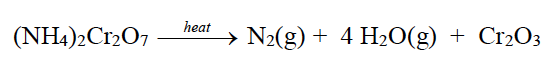Calculate:

(i) the quantity in moles of (NH4)2Cr2O7 if 63 gm of (NH4)2Cr2O7 is heated.

(ii) the quantity in moles of nitrogen formed.

(iii) the volume in litres or dm3 of N2 evolved at S.T.P.

(iv) the mass in grams of Cr2O3 formed at the same time.

[Atomic masses: H=1, Cr= 52, N=14]

(b) (i) For each of the substances listed below, describe the role played in the extraction of aluminium.

1. Cryolite
2. Sodium hydroxide
3. Graphite

(ii) Explain why:

1. In the electrolysis of alumina using the Hall Heroult’s Process the electrolyte is covered with powdered coke.
2. Iron sheets are coated with zinc during galvanization.

(a) i. 252 ÷ 63 or 0.25 moles

ii. 0.25 moles of N2

iii. 0.25 x 22.4 l or 5.6 l

iv. Mass of salt = 0.25 x 152 = 38 g

(b) (i)

1. Cryolite is used to reduce the temperature to melt the (purified ore) / to increase the conductivity / it acts as a solvent.
2. Sodium hydroxide or NaOH is used to dissolve bauxite / to concentrate the ore / insoluble impurities get precipitated or removed/ NaOH is used to dissolve aluminium oxide or Al2O3 / to convert bauxite to sodium aluminate / to purify bauxite.
3. Graphite lining of the electrolytic tank is used as cathode / graphite rods are used as anode.

(ii)

1. Coke powder does not allow the radiation of heat from the electrolyte/ prevents oxidation or burning of the anode / prevents loss of heat.
2. Zinc is more reactive than iron / zinc is more electropositive than iron / Zinc is higher in the activity series / to prevent rusting / corrosion.

### Question 6:

(a) (i) Give balanced chemical equations for the action of sulphuric acid on each of the following:

1. Potassium hydrogen carbonate.
2. Sulphur.

(ii) In the contact process for the manufacture of sulphuric acid give the equations for the conversion of sulphur trioxide to sulphuric acid.

(b) (i) Copy and complete the following table:

 Anode Electrolyte Purification of Copper

(ii) Write the equation taking place at the anode.

(c) Explain the following:

(i) Dilute nitric acid is generally considered a typical acid but not so in its reaction with metals.

(ii) Concentrated nitric acid appears yellow when it is left standing in a glass bottle.

(iii) An all glass apparatus is used in the laboratory preparation of nitric acid.

(a) (i)

1. 2KHCO3 + H2SO4 → K2SO4 + 2H2O + 2CO2
2. S + 2H2SO4 → 3SO2 + 2H2O

(ii)

1. SO3 + H2SO4 → H2S2O7
2. H2S2O7 + H2O → 2 H2SO4

(b) (i)

 Anode Electrolyte Purification of Copper Impure copper metal Copper sulphate CuSO4 any soluble salt of copper

(ii) Cu → Cu 2+ + 2e or

Cu – 2e- → Cu2+

(c) (i) Dilute nitric acid also has oxidizing properties does not form H2 with all metals except Mg & Mn / produces oxides of nitrogen

(ii) Conc. HNO3 decomposes to form nitrogen dioxide which dissolves in it.

Or

Conc. HNO3 produces reddish brown gas which dissolves in it.

(iii) HNO3 vapours are very corrosive in nature / they react with rubber or cork / they attack or damage or corrode or destroy any other material.

### Question 7:

(a) The following questions are pertaining to the laboratory preparation of hydrogen chloride gas:

(i) Write the equation for its preparation mentioning the condition required.

(ii) Name the drying agent used and justify your choice.

(iii) State a safety precaution you would take during the preparation of hydrochloric acid.

(b) An element L consists of molecules.

(i) What type of bonding is present in the particles that make up L?

(ii) When L is heated with iron metal, it forms a compound FeL. What chemical term would you use to describe the change undergone by L?

(c) From the list of the following salts choose the salt that most appropriately fits the description given in the following:

[AgCl, MgCl2, NaHSO4, PbCO3, ZnCO3, KNO3, Ca(NO3)2]

(i) A deliquescent salt.

(ii) An insoluble chloride.

(iii) On heating, this salt gives a yellow residue when hot and white when cold.

(iv) On heating this salt, a brown coloured gas is evolved.

(a) (i)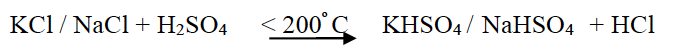P2O5 and CaO react with HCl vapours, H2SO4 does not

(ii) Concentrated sulphuric acid or H2SO4 is used as the drying agent.

(iii) Funnel arrangement as HCl is highly soluble in water and to avoid back suction

(b) (i) Covalent bonding / covalent bond

(ii) L is getting reduced

(c) (i) MgCl2

(ii) AgCl

(iii) ZnCO3

(iv) Ca(NO3)2

The ICSE Class 10 Chemistry Question Paper Solution 2015 must have helped students with their exam preparation. The answers in the Solution pdf have provided an in-depth to 2015 questions and the way answers to be written to score high marks in the board exam. Apart from the 2015 paper solution, students can find the answers to other papers of ICSE Class 10 Previous Years Questions by clicking here. Happy Learning and stay tuned to BYJU’S for the latest update on ICSE/CBSE/State Boards/Competitive exams. Also, don’t forget to download the BYJU’S App.